# Lab 7 - LR Circuits

## Introduction

The English physicist Michael Faraday found in 1831 that when the current through a coil changes, the coil produces a changing magnetic field (in addition to the field of the changing current), which induces an electromotive force ("emf") in the coil itself. In 1834, the German physicist Heinrich Lenz refined this further by showing that the induced current driven by this emf will be in the direction that opposes the change in the original current. We call this phenomenon self-induction, and the coils are called inductors. At the time that Faraday announced his discovery, he was asked what possible use such knowledge could be? His reply was: "Of what use is a newborn baby?" As happens with many seemingly arcane discoveries, Faraday's investigations of induction led to several common and useful modern electrical devices. Inductors, like capacitors, affect the time characteristics of an AC (alternating current) circuit and are, therefore, used to tune radio circuits, filter out unwanted noise, etc. The telephone receiver makes use of a type of inductor, as do stereo speaker systems and microphones. In this lab you will examine the effect of an inductor on the current and voltage in a simple circuit.

## Discussion of Principles

The inductance of a circuit, usually symbolized by L, and measured in henry (H), is the tendency of a circuit to oppose any changes in the current. This opposition to a change in the current shows up as a slowing of the rise or fall of the current in circuits. Inductance is a property of electrical devices. Devices having this property are called inductors. The inductance of a device, like resistance and capacitance, depends on geometrical factors like the size of the device and on the material from which the device is made. It does not depend on the current in the device. Consider a simple circuit consisting of a switch, a resistor R, and a battery. When the switch is closed, the current I in the circuit will increase very quickly to a steady value given by Ohm's Law,
I = ΔV / R
where
ΔV
is the voltage or emf of the battery. Consider the same circuit with the addition of an inductor, as shown in Fig. 1.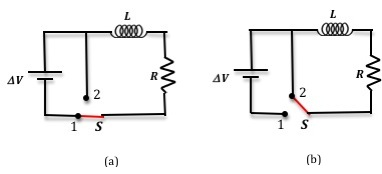Figure 1: LR circuit

When the switch is in position 1 as shown in Fig. 1(a), the rising current produces a rising magnetic flux in the inductor. This induced magnetic flux produces an electromotive force (emf) that is of opposite polarity to that of the battery, which results in an induced current opposing the current from the battery. The current, therefore, rises more slowly than it would otherwise have done without the inductor. How much more slowly the current increases depends on the size of the inductance and the value of the resistance. Even though the time it takes the current to rise to its final value is longer, the actual final value is the same as that which would be achieved without the inductor. Consider the situation where the switch has been in position 1 for sometime and a steady current is flowing in the circuit. If the switch is now moved to position 2 as in Fig. 1(b) the battery is no longer part of the circuit. The inductor will prevent the current from instantly dropping to zero. Just as the inductor resisted a rapid increase in current, it also resists a rapid decrease in current. For the circuit shown in Fig. 1(a), Kirchhoff's loop equation can be written as
( 1 )
ΔVIRL
 dI dt
= 0.

The solution to Eq. (1) is
( 2 )
I = If1 − e(−R/L)twhere If represents the final steady current flowing in the circuit after an infinite length of time, R is the circuit resistance, and L is the inductance of the coil. From this expression you can see that current increases exponentially. This is shown in Fig. 2(a). When the switch is moved to position 2, for the circuit shown in Fig. 1(b), Kirchhoff's loop equation is now given by
( 3 )
IRL
 dI dt
= 0.

The solution to Eq. (3) is
( 4 )
I = I0e(−R/L)t
where I0 represents the initial maximum current in the circuit at t = 0. You can see from this expression that the current decreases exponentially. See Fig. 2(b).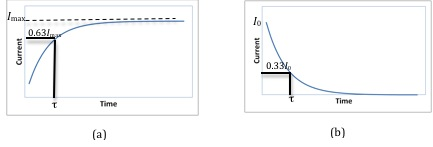Figure 2: Current versus time graph

### Time Constant

The mathematical analysis of a simple LR circuit is similar to that of a simple RC circuit (a circuit consisting of a resistor and a capacitor in series). In an RL circuit, the time constant
τ
is defined by
( 5 )
τ = L/R
The time constant is also defined as the amount of time it takes the current to reach 63% or
(1 − e−1
of its final value. Note that
Eq. (2) has the same form as the equation describing the charging of a capacitor.
Since the current is changing with time, the potential difference across the resistor must also be changing with time. The equation for the potential difference across the resistor is obtained by using Ohm's Law,
ΔV = IR
( 6 )
ΔVR = ΔVf1 − e(−t/τ)where
ΔVf
is the final or maximum potential difference across the resistor and is equal to the emf of the battery.
Note that at
t = 0, (1−et/τ) = 0
and the current through the circuit and the potential difference across the resistor are zero. Therefore, the potential drop is entirely across the inductor. At t = infinity (a long time after the switch has been in position 1),
(1 − et/τ) = 1
and the current through the circuit and the potential difference across the resistor are maximum at If and
ΔVR
Therefore, the potential drop is entirely across the resistor and the potential drop across the inductor is zero.
Without the inductor in the circuit the current in the resistor would drop very quickly to zero once the switch is moved to position 2. When the inductor is in the circuit, it opposes this change in current, and so the current drops more slowly. The current and voltage across the resistor t seconds after the battery is removed from the circuit by moving the switch to position 2 are given by the following.
( 7 )
I = I0e(−t/τ)
( 8 )
ΔVR = ΔV0e(−t/τ)
Here
τ
is the time needed for the current to decrease to 33% of its original value at t = 0.
Consider Eq. (7) and Eq. (8). At
t = 0, et/τ = 1,
and the current through the circuit and the potential difference across the resistor are maximum. Therefore, the potential drop is entirely across the resistor and the potential drop across the inductor is zero. At t = infinity (a long time after the switch has been in position 2),
et/τ = 0,
and the current through the circuit and the potential difference across the resistor are zero. Therefore, the potential drop is entirely across the inductor and the potential drop across the resistor is zero.
At any given time t the sum of the potential drops across the resistor and the inductor will be equal to the emf of the battery.
( 9 )
emfbattery = ΔVR + ΔVL
The potential difference
ΔVR
across the resistor as a function of time is shown here in Fig. 3 and Fig. 4 shows the voltage drop across the inductor as a function of time. Note that when the voltage across the resistor is a maximum, the voltage across the inductor is zero and vice versa as discussed earlier.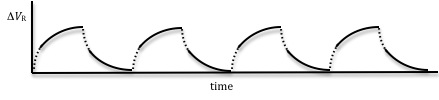Figure 3: Voltage across the resistor as a function of time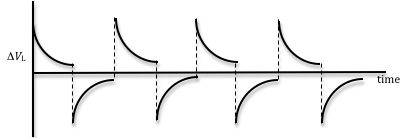Figure 4: Voltage across the inductor as a function of time

( 10 )
 ΔVf − ΔVR ΔVf
= et (R/L)
where
τ
has been replaced with L/R. Taking the natural log of both sides of this equation and multiplying by –1, we get
( 11 )
−ln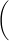ΔVf − ΔVR ΔVf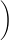=
 R L
t.

If you consider the left side of the equation to be a single variable, say y, then the equation becomes
y = (R/L)t
which is a linear equation of the form
y = mx
The inductance can be determined from the slope of this line.
Similarly, Eq. (8) can be written as
( 12 )
−lnΔVR ΔV0=
 R L
t.

A plot of
−lnΔVR ΔV0versus time t for a decreasing current (soon after the switch is opened) will give a straight line with a slope of R/L from which the inductance can be determined.

### Using a Square Wave to Simulate the Role of a Switch

In this experiment, rather than using a switch, we will be using a signal generator that can generate periodic wave forms of varying shapes, like a sine wave, a triangular wave, and a square wave. Both the frequencies and amplitudes of the wave forms can also be adjusted. Here we will use the signal generator to produce a time-varying voltage, with a square wave form, across the inductor similar to the one shown in Fig. 5.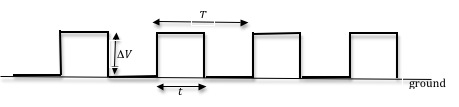Figure 5: A square wave with period T

The output voltage from the signal generator changes back and forth from a constant positive value to a constant zero volts in equal intervals of time t. The time
T = 2t
is the period of the square wave. During the first half of the cycle, when the voltage is positive, it is similar to the switch being in position 1. During the second half of the cycle, when the voltage is zero, it is the same as the switch being in position 2. So the square wave, which is a DC voltage that is turned on and off periodically, serves as both battery and switch in the setup in Fig. 1.
The signal generator allows this switching to be done repeatedly, and it is possible to optimize the data collection by adjusting the frequency of the repetition. This frequency will depend on the time constant of the RL circuit. When the time t is larger than the time constant
τ
of the RL circuit, the current in the circuit will have enough time to reach the steady state and the voltage across the inductor will be as shown in Fig. 4.

## Objective

The objective of this experiment is to examine the dynamic behavior of an LR circuit by using an oscilloscope to visualize the voltage across the resistor for both rising and decreasing current. You will also determine the time constant and inductance of the coil.

## Equipment

• PASCO circuit board
• Capstone software
• Signal interface with power output
• Connecting wires
• Multimeter

## Procedure

Please print the worksheet for this lab. You will need this sheet to record your data.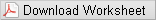### Setting Up the LR Circuit

The RLC circuit board that you will be using consists of three resistors and one inductance coil among other elements. The value of the inductor can be changed by inserting an iron core into the coil. See Fig. 6 below. In theory you can, therefore, have different combinations of resistors and capacitors. In this experiment you will use the 10-Ω resistor and the inductor coil.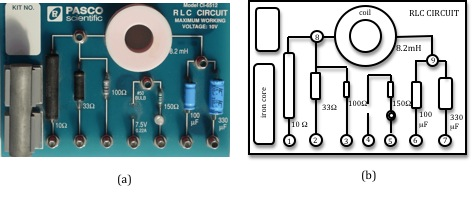Figure 6: RLC circuit board

1
Connect the far right output terminal of the signal interface to the inductor at point 9.
2
Connect point 1 to the second output terminal of the signal interface to complete the circuit.
3
Connect the voltage probe into analog channel A.
4
To measure the voltage across the resistor, connect one lead of the voltage probe to point 8 and the other lead to point 1. Make sure that the ground of the interface (the "–" lead) is connected to the same side of the resistor as the ground of the signal generator (power output). Your circuit connection should look like that in Fig. 7.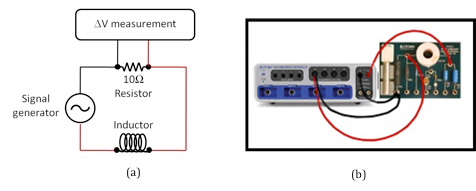Figure 7: Circuit diagram

Checkpoint 1:

### Procedure A: Determining L from Time Constant

The computer will function as the oscilloscope to record
ΔVR
and as the signal generator.
5
Open the Capstone file associated with this lab. A screen similar to Fig. 8 is displayed.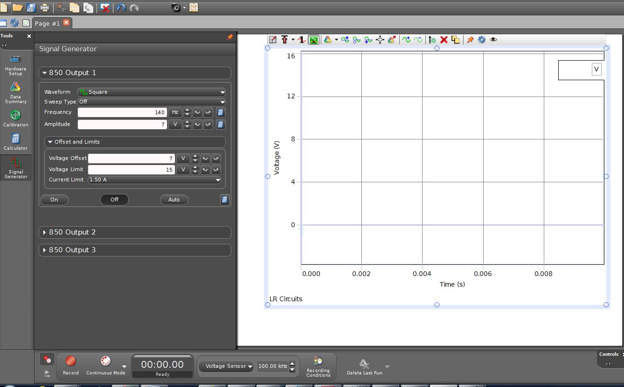Figure 8: Opening screen of LR circuit file

6
The file should open with the signal generator set to produce a positive square wave.
7
If not already set, set the voltage to 7-V amplitude with the frequency at any value between 120 and 180 Hz and set the voltage offset to 7 V.
8
Turn on the signal generator by clicking ON in the signal generator window, and monitor the signal by clicking MONITOR in the main window. There should be a signal trace like that shown in Fig. 9. This will allow you to observe how the voltage on the resistor varies as a function of time. Click STOP after a few seconds. The data will remain in the scope window until the next time the START button is clicked.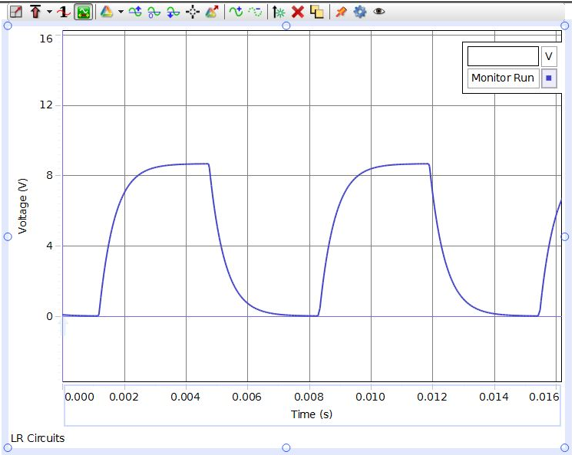Figure 9: A sample signal

9
Adjust the voltage (potential difference) and time scales so that about one wavelength is displayed in the scope window, by placing the cursor on the values of each scale and dragging left-right or up-down as appropriate.
10
Select the Show Coordinates button from the buttons above the graph. See Fig. 10.
11
Using Show Coordinates, determine the starting time (i.e., when the potential difference begins to increase from 0 volts) and record it on the worksheet.
12
Calculate 63% of the maximum potential difference (0.63
ΔVf).
13
Use Show Coordinates to determine the time at which that potential difference occurs. Record this time on the worksheet.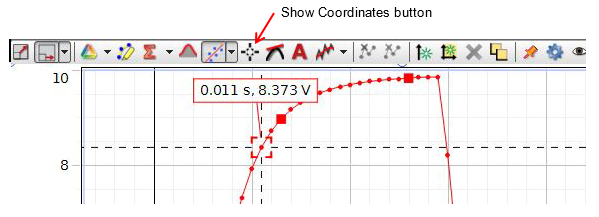Figure 10: Show Coordinates

14
From the two time values obtained in steps 11 and 13, determine and record the time required for the signal to go from
ΔVR = 0 to ΔVR = 0.63 ΔVf
This is your experimental value for the time constant
τ
15
Use a multimeter to measure the combined resistance of the coil and resistor in series. This is the total resistance of the circuit. See Appendix K. To do this, remove any other wire leads from the PASCO circuit board and then connect the multimeter around the resistor and inductor combination, as shown in Fig. 11.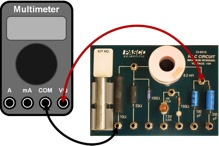Figure 11: Physical wiring to measure total resistance of RL circuit

16
Calculate the experimental value of the inductance using Eq. (5) and the experimental values of
τ
and R. Record this value on the worksheet.
17
Use the inductance value printed next to the inductor on the PASCO circuit board as the accepted value, and record this on the worksheet.
18
Calculate the percent error between the experimental and accepted values of the inductance, and record it on the worksheet. See Appendix B.
Checkpoint 2:

### Procedure B: Measuring Voltage for Increasing Current

19
From the recorded oscilloscope trace, measure the voltage
ΔVR
across the resistor and the time t for six points on the rising part of the curve. Record these values in Data Table 1.
20
From the final potential difference and the values of
ΔVR
that you just recorded, calculate the quantities for the remaining two columns in Data Table 1.
21
Use Excel to plot
−ln[(ΔVfΔVR)/ΔVf
versus t for the six points. See
Appendix G.
22
Using the trendline option in Excel to draw the best fit line to your data, determine the slope of the line. See Appendix H. Record this on the worksheet.
23
Use the slope value to find the inductance and record this on the worksheet.
24
Calculate the percent error between the accepted value of the inductance and the value obtained from the slope of the graph. Record this value on the worksheet.
Checkpoint 3:

### Procedure C: Measuring Voltage for Decreasing Current

25
From the recorded oscilloscope trace, measure the voltage
ΔVR
across the resistor and the time t for six points on the falling part of the curve. Record these values in Data Table 2.
Note that
ΔV0
for the falling part of the curve is the same as
ΔVf
for the rising part of the curve.
26
From the initial potential difference
ΔV0
and the values of
ΔVR
that you just recorded, calculate the quantities for the remaining two columns in Data Table 2.
27
Use Excel to plot
−ln[(ΔVR)/ΔV0
versus t for your six points.
28
Using the trendline option in Excel to draw the best fit line to your data, determine the slope of the line and record this value on the worksheet.
29
From the slope value find the inductance and record this on the worksheet.
30
Calculate the percent error between the accepted value of the inductance and the value obtained from the slope of
−ln[(ΔVR)/ΔV0
versus t graph. Record this value on the worksheet.
Checkpoint 4: